# Presentation on metrology.pptx

28. May 2023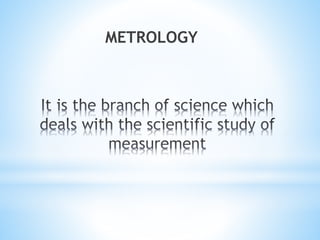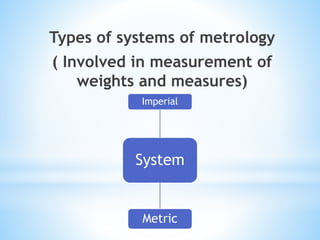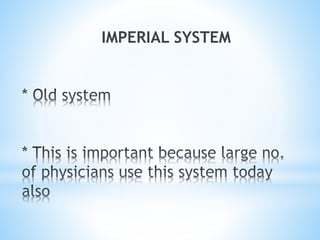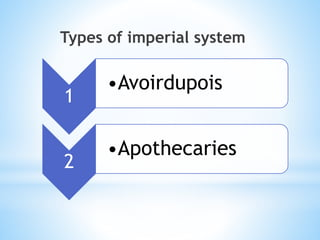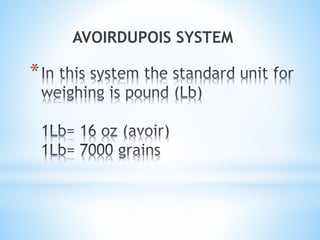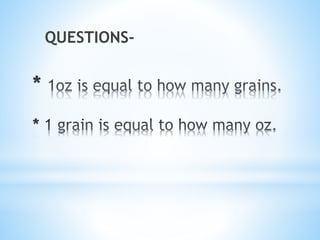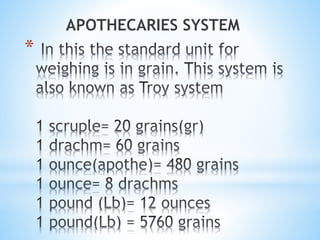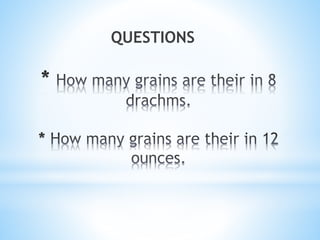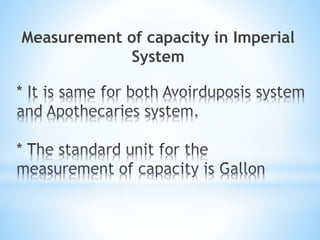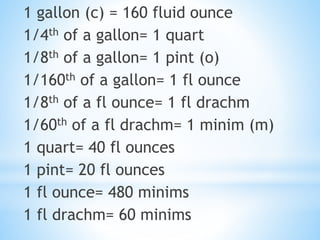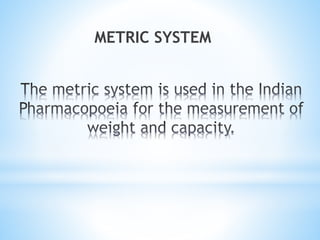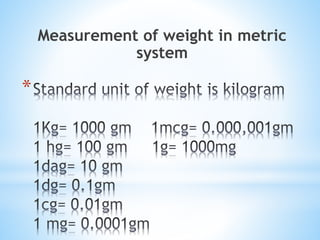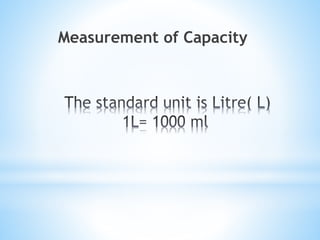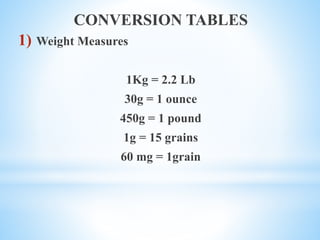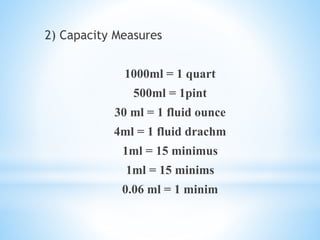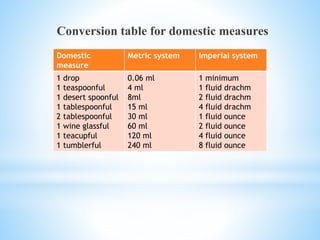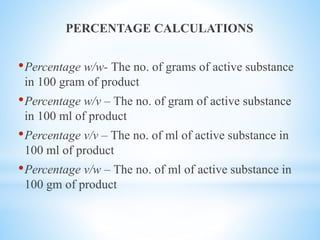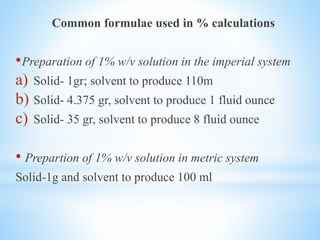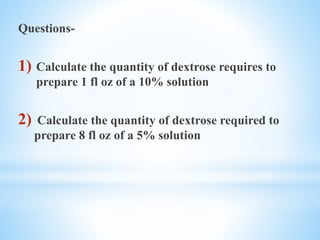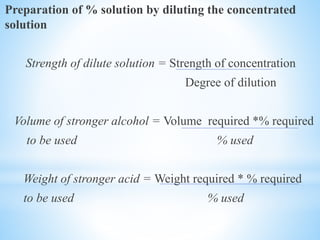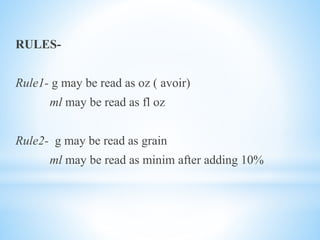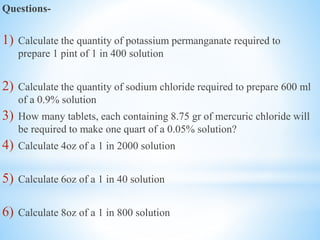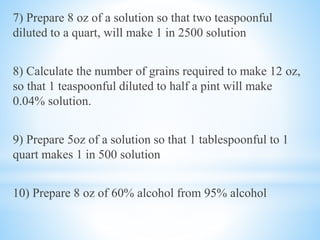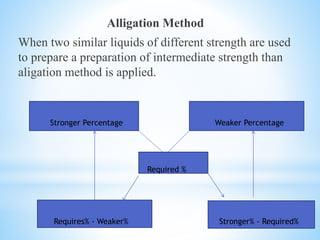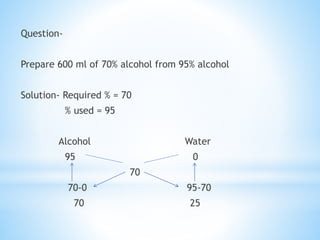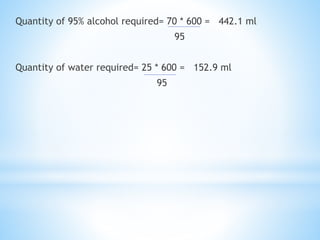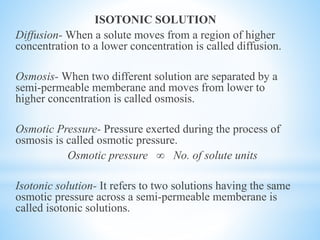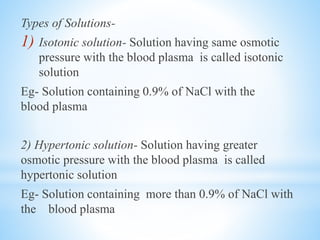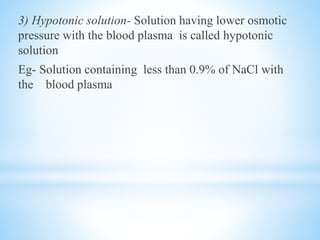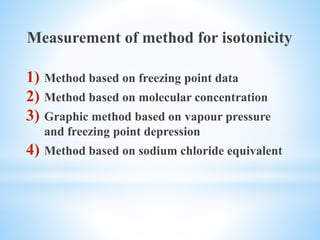1 von 32

### Presentation on metrology.pptx

• 3. Types of systems of metrology ( Involved in measurement of weights and measures) System Imperial Metric
• 5. Types of imperial system 1 •Avoirdupois 2 •Apothecaries
• 10. Measurement of capacity in Imperial System
• 11. 1 gallon (c) = 160 fluid ounce 1/4th of a gallon= 1 quart 1/8th of a gallon= 1 pint (o) 1/160th of a gallon= 1 fl ounce 1/8th of a fl ounce= 1 fl drachm 1/60th of a fl drachm= 1 minim (m) 1 quart= 40 fl ounces 1 pint= 20 fl ounces 1 fl ounce= 480 minims 1 fl drachm= 60 minims
• 13. * Measurement of weight in metric system
• 15. CONVERSION TABLES 1) Weight Measures 1Kg = 2.2 Lb 30g = 1 ounce 450g = 1 pound 1g = 15 grains 60 mg = 1grain
• 16. 2) Capacity Measures 1000ml = 1 quart 500ml = 1pint 30 ml = 1 fluid ounce 4ml = 1 fluid drachm 1ml = 15 minimus 1ml = 15 minims 0.06 ml = 1 minim
• 17. Conversion table for domestic measures Domestic measure Metric system Imperial system 1 drop 1 teaspoonful 1 desert spoonful 1 tablespoonful 2 tablespoonful 1 wine glassful 1 teacupful 1 tumblerful 0.06 ml 4 ml 8ml 15 ml 30 ml 60 ml 120 ml 240 ml 1 minimum 1 fluid drachm 2 fluid drachm 4 fluid drachm 1 fluid ounce 2 fluid ounce 4 fluid ounce 8 fluid ounce
• 18. PERCENTAGE CALCULATIONS •Percentage w/w- The no. of grams of active substance in 100 gram of product •Percentage w/v – The no. of gram of active substance in 100 ml of product •Percentage v/v – The no. of ml of active substance in 100 ml of product •Percentage v/w – The no. of ml of active substance in 100 gm of product
• 19. Common formulae used in % calculations •Preparation of 1% w/v solution in the imperial system a) Solid- 1gr; solvent to produce 110m b) Solid- 4.375 gr, solvent to produce 1 fluid ounce c) Solid- 35 gr, solvent to produce 8 fluid ounce • Prepartion of 1% w/v solution in metric system Solid-1g and solvent to produce 100 ml
• 20. Questions- 1) Calculate the quantity of dextrose requires to prepare 1 fl oz of a 10% solution 2) Calculate the quantity of dextrose required to prepare 8 fl oz of a 5% solution
• 21. Preparation of % solution by diluting the concentrated solution Strength of dilute solution = Strength of concentration Degree of dilution Volume of stronger alcohol = Volume required *% required to be used % used Weight of stronger acid = Weight required * % required to be used % used
• 22. RULES- Rule1- g may be read as oz ( avoir) ml may be read as fl oz Rule2- g may be read as grain ml may be read as minim after adding 10%
• 23. Questions- 1) Calculate the quantity of potassium permanganate required to prepare 1 pint of 1 in 400 solution 2) Calculate the quantity of sodium chloride required to prepare 600 ml of a 0.9% solution 3) How many tablets, each containing 8.75 gr of mercuric chloride will be required to make one quart of a 0.05% solution? 4) Calculate 4oz of a 1 in 2000 solution 5) Calculate 6oz of a 1 in 40 solution 6) Calculate 8oz of a 1 in 800 solution
• 24. 7) Prepare 8 oz of a solution so that two teaspoonful diluted to a quart, will make 1 in 2500 solution 8) Calculate the number of grains required to make 12 oz, so that 1 teaspoonful diluted to half a pint will make 0.04% solution. 9) Prepare 5oz of a solution so that 1 tablespoonful to 1 quart makes 1 in 500 solution 10) Prepare 8 oz of 60% alcohol from 95% alcohol
• 25. Alligation Method When two similar liquids of different strength are used to prepare a preparation of intermediate strength than aligation method is applied. Stronger Percentage Weaker Percentage Required % Requires% - Weaker% Stronger% - Required%
• 26. Question- Prepare 600 ml of 70% alcohol from 95% alcohol Solution- Required % = 70 % used = 95 Alcohol Water 95 0 70 70-0 95-70 70 25
• 27. Quantity of 95% alcohol required= 70 * 600 = 442.1 ml 95 Quantity of water required= 25 * 600 = 152.9 ml 95
• 28. ISOTONIC SOLUTION Diffusion- When a solute moves from a region of higher concentration to a lower concentration is called diffusion. Osmosis- When two different solution are separated by a semi-permeable memberane and moves from lower to higher concentration is called osmosis. Osmotic Pressure- Pressure exerted during the process of osmosis is called osmotic pressure. Osmotic pressure ∞ No. of solute units Isotonic solution- It refers to two solutions having the same osmotic pressure across a semi-permeable memberane is called isotonic solutions.
• 29. Types of Solutions- 1) Isotonic solution- Solution having same osmotic pressure with the blood plasma is called isotonic solution Eg- Solution containing 0.9% of NaCl with the blood plasma 2) Hypertonic solution- Solution having greater osmotic pressure with the blood plasma is called hypertonic solution Eg- Solution containing more than 0.9% of NaCl with the blood plasma
• 30. 3) Hypotonic solution- Solution having lower osmotic pressure with the blood plasma is called hypotonic solution Eg- Solution containing less than 0.9% of NaCl with the blood plasma
• 31. Measurement of method for isotonicity 1) Method based on freezing point data 2) Method based on molecular concentration 3) Graphic method based on vapour pressure and freezing point depression 4) Method based on sodium chloride equivalent